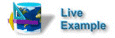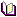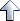Application Techniques

# Manipulating Strings with JavaScript

How to use JavaScript to perform basic string manipulation operations.

Details

Category

DescriptionYou can run this technique code from:Related reading

See the chapter on advanced page topics in the Programmer's Guide

# Replacing strings```  Jack's birthday is 8/22
```

To replace the string Jack with the string Jacques, you can use the JavaScript `replace()` method:

```  <script>
<!--
demoString = "Jack's birthday is 8/22";

// This is the replace() method.  It is invoked on
// a string and takes two parameters:
//   1) piece to substitute out
//   2) piece to substitute in
// You can put regular expression patterns in the replace
// method as parameters.  In many cases, this is what you
// would do because you don't always know what the starting
// string is exactly.

newString = demoString.replace("Jack","Jacques");

// This line just prints the output to the page.

document.write("<pre>   " + newString + "</pre>");
//-->
</script>
```

The result is:

```  Jacques's birthday is 8/22
```

# Inserting stringsTo insert the string also after the string is, you can use the JavaScript `indexOf()` and `substring()` methods:

```  <script>
<!--
demoString = "Jack's birthday is 8/22";
newString = demoString.replace("Jack","Jacques");

// The indexOf() method returns a number where
// the parameter you give it first appears in the
// string it is invoked on.  In the word SilverStream,
// indexOf("S") would return 0, indexOf("i") would
// return 1, and indexOf("a") would return 10.

whereIS = newString.indexOf("is");

// The substring() method returns a new string that's
// a piece of the string that substring() is invoked on.
// substring() takes either 1 or 2 parameters:
//   1) starting point for new string
//   2) ending point for new string (length of new string)
// If no ending point is specified, the new string goes from
// the starting point until the end.
//
// ** Important: When you specify an ending point,
// substring returns up to but not including the character
// at the ending point.  For instance, take this string:
//      someString = "Color is red";
//
//    someString.substring(0,4) would return 'Colo'
//
//    C = 0      <-- included
//    o = 1      <-- included
//    l = 2      <-- included
//    o = 3      <-- included
//    r = 4
//
// The second parameter does not map to the index of a character
// in a string.  It specifies how long the new string should be.

firstPart = newString.substring(0,whereIS + 2);
lastPart = newString.substring(whereIS + 3);
newString = firstPart + " also " + lastPart;
document.write("<pre>   " + newString + "</pre>");
//-->
</script>
```

Or, you can use the JavaScript `replace()` method:

```  <script>
<!--
demoString = "Jack's birthday is 8/22";
newString = demoString.replace("Jack","Jacques");
newString = newString.replace("is","is also");
document.write("<pre>   " + newString + "</pre>");
//-->
</script>
```

Either way, the result is:

```  Jacques's birthday is also 8/22
```

# Extracting stringsTo extract the string containing the date (month and day), you can use the JavaScript `substring()` method:

```  <script>
<!--
demoString = "Jack's birthday is 8/22";

// This takes a substring from the 4th to last character
// in the string until the end.  The length property
// returns a number equal to, as its name implies, the length
// of the string.

birthday = demoString.substring(demoString.length - 4);
document.write("<pre>   " + birthday + "</pre>");
//-->
</script>
```

The result is:

```  8/22
```

To extract the string containing just the day, you can use the JavaScript `substring()` and `lastIndexOf()` methods:

```  <script>
<!--
demoString = "Jack's birthday is 8/22";

// Similar to indexOf(), lastIndexOf() returns
// the position of the character at its last
// occurrence in the string.
//      someString = "SilverStream";
//      someIndex = someString.lastIndexOf("S");
// someIndex would be equal to 6

dayNumber = demoString.substring(demoString.lastIndexOf("/") + 1);
document.write("<pre>   " + dayNumber + "</pre>");
//-->
</script>
```

The result is:

```  22
```